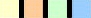.net                                       HOME  |  GALLERIES  |  E-ZINE  |  RESOURCES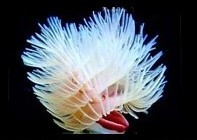________________________

1  2  0     G  A  L  L  O  N

M  i  x  e  d         R  e  e  f

________________________

 S O F T    C O R A L S

 L P S   C O R A L S

 S P S   C O R A L S

 M U S H R O O M S,  E T C.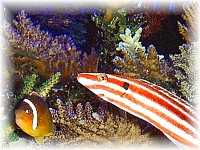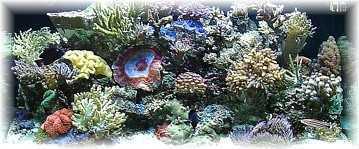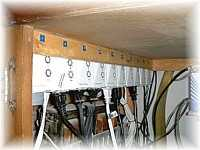F I S H E S

 F U L L    T A N K    P H O T O

 E Q U I P M E N T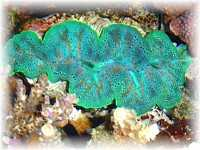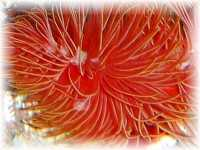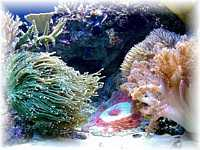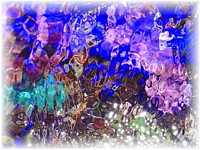C L A M S

 M I S C.   I N V E R T S

 A R E A     S H O T S

 S P E C I A L

HOME  |  GALLERIES  |  E-ZINE  |  RESOURCES